You are viewing documentation for an older version. For current documentation - click here.

FusionWidgets XT accepts gradient mix formula for many elements in different charts.

For example, in an angular gauge, you can set a gradient mix formula for the color scale, pivot etc. In a horizontal linear gauge, you can again set a gradient mix formula for the color scale. The gradient mix for the color scale in an angular gauge is set using the gaugeFillMix=’{light-10},{light-70},{dark-10}’.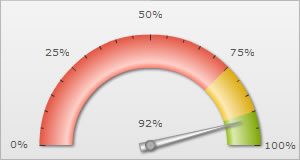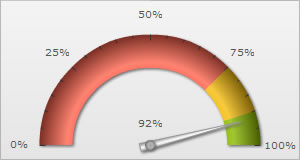Chart with the default gradient mix for the color scale Chart with the gradient mix set by for the color scale
Here, we explain how you can build the formula for complex gradient formations.

• It takes a base color for fill and manipulates it to achieve light and dark color combinations.
• From the base color, you can derive lighter and darker shades of the color by specifying a percentage between 0-100 (as we’ll see next)
• You can include other colors (not related to base color) by directly entering their hex code in the formula.
• You can also include the base color itself in the gradient formation using the word color.
• Each color variation has to be necessarily enclosed between parenthesis {}
• The color variations have to be necessarily separated by comma.

Examples:

• {light-10},{light-70},{dark-10},{color}
light-10 represents a color which is 10% lighter than the color originally present there. Suppose you have an angular gauge chart having 3 color ranges, one red, one green and one yellow. So when the gauge comes across this formula (which lets say we have applied to the color scale), the color scale will be filled with a color which is 10% lighter than the color present there originally - in the red part of the color range, the color will be 10% lighter than the red color defined for it, in the green part of the color range, it will be 10% lighter than the green color defined there and so on. Similarly, light-70 and dark-10 represents a color 70% lighter and 10% darker than the color originally present and color represents the color which is originally present over there.

• {light-10},{light-20},{light-60},{FFFFFF},{dark-30},{dark-40},{dark-40}
In this gradient mix, as soon as FFFFFF is encountered, the color scale will be filled with FFFFFF irrespective of the color originally present over there.

Below are how the angular gauges will look as with the above gradient formulas applied to their color scales: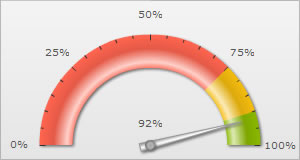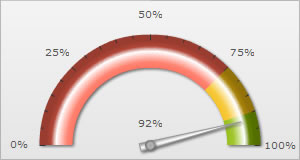{light-10},{light-70},{dark-10},{color} {light-10},{light-20},{light-60},{FFFFFF},{dark-30},{dark-40},{dark-40}
To sum up, the gradient fill mix formula can take any of the following literals:
• {dark-xx} – where xx represents the percentage of darkness w.r.t base color
• {light-xx} – where xx represents the percentage of lightness w.r.t base color
• {color} – Actual base color
• {HexCode} like {FFFFFF} or {FF0000}

Specifying ratio

Apart from specifying the color mix, you can also specify the ratio of each color in the mix. The ratio determines how far that particular color will spread before mixing with the next color and forming a gradient.

Ratio is specified by separating them by commas e.g.,
<chart gaugeFillMix='{light-10},{light-70},{dark-10}' gaugeFillRatio='60,20,20'…>

The ratios should sum up to 100 and the number of ratios should be equal to the number of colors you have specified in the gradient mix. FusionWidgets XT will automatically map each ratio to the corresponding color in the gradient mix.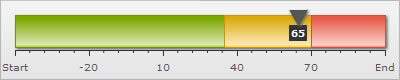Horizontal linear gauge with <chart ... gaugeFillMix='{light-10},{light-70},{dark-10}'...>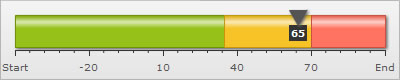<chart gaugeFillMix='{light-10},{light-70},{dark-10}' gaugeFillRatio='60,20,20'…>